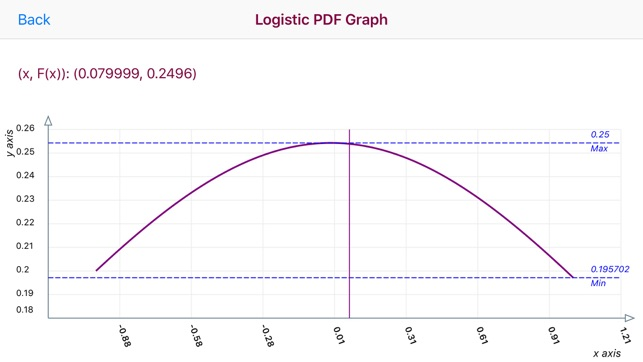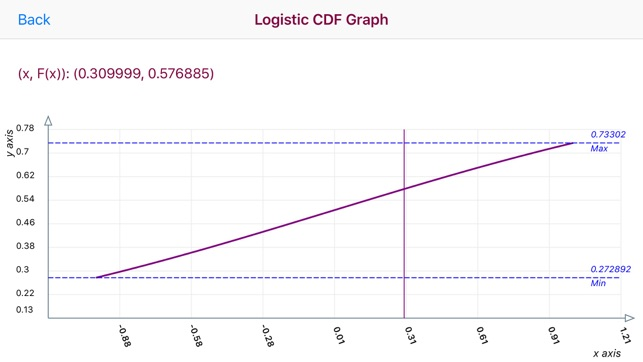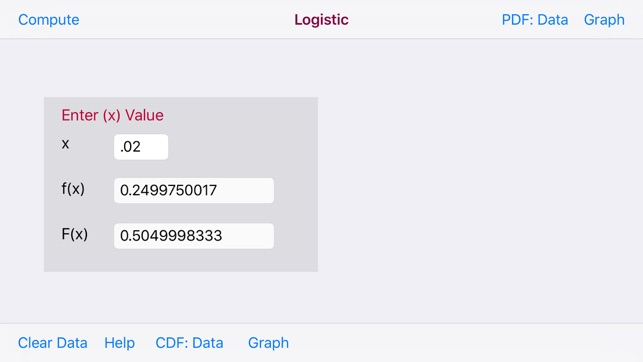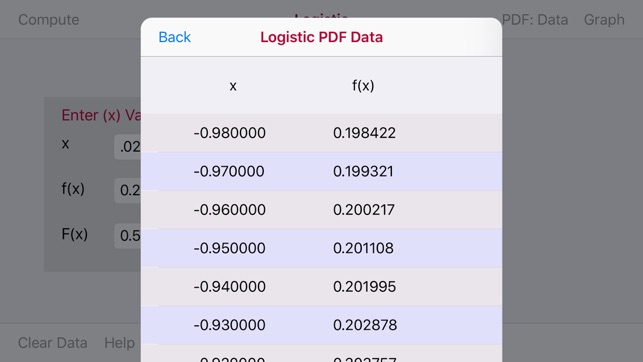Screenshots

•••••Description

The Logistic Distribution is a continuous single-parameter distribution which has been used extensively to represent growth functions. The Logistic Distribution curve has a shape similar to the Normal Distribution.

The Logistic Disribution Calculator accepts a random variable x and returns the probability that the random variable with a standard Logistic Distribution has a value less than variable x. The Logistic Distribution F(x) graph displays the Logistic Distribution as a bell-shaped curve for a given value of the random variable x.

The Data Tables and Graphs increment the x value 200 times with a 0.01 increment value with -1.0 <= x <= 1.0.

The graphs are touch enabled. Upon touching the graph a vertical line appears. Move the vertical line to the left or right to display the point (x/f(x)) data in the upper left of the graph.

The horizontal x-axis displays x values. The vertical y-axis plots a range of F(x) values.

Horizontal Max and Min dashed line display the Maximum and Minimum F(x) values

What’s New

Version 1.5

Upgrade to iOS 12

Information

Seller
Donald Schaefer
Size
8 MB
Category
Education
Compatibility

Requires iOS 12.0 or later. Compatible with iPhone, iPad, and iPod touch.

Languages

English

Age Rating
Rated 4+
© 2014 Donald Schaefer
Price
Free

Supports

•